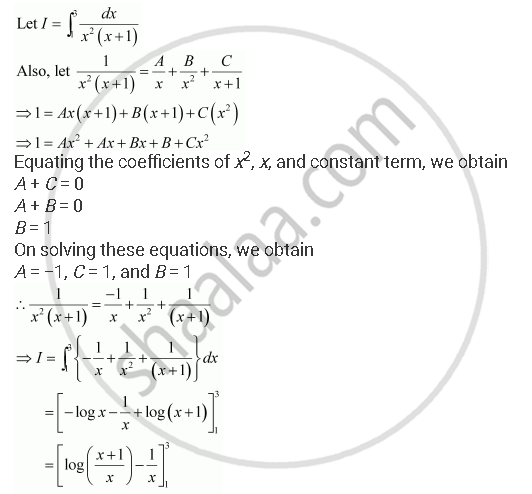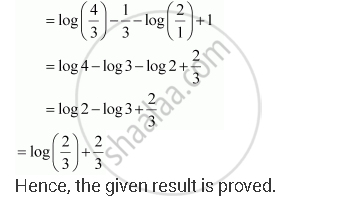Share
Notifications

View all notifications
Books Shortlist
Your shortlist is empty

# Solution for Prove the Following Int_1^3 Dx/(X^2(X +1)) = 2/3 + Log 2/3 - CBSE (Science) Class 12 - Mathematics

Login
Create free account

Forgot password?
ConceptDefinite Integral as the Limit of a Sum

#### Question

Prove the following int_1^3 dx/(x^2(x +1)) = 2/3 + log 2/3

#### SolutionIs there an error in this question or solution?

#### Video TutorialsVIEW ALL 

Solution Prove the Following Int_1^3 Dx/(X^2(X +1)) = 2/3 + Log 2/3 Concept: Definite Integral as the Limit of a Sum.
S